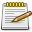# GetWaterVertexPosition

(diff) ← Older revision | Latest revision (diff) | Newer revision → (diff)Script Example Missing Function GetWaterVertexPosition needs a script example, help out by writing one. Before submitting check out Editing Guidelines Script Examples.

Gets the world position of a vertex (i.e. corner) of a water area. Each water area is either a triangle or quad (rectangle) so each has 3 or 4 corners.

## Syntax

`int int float getWaterVertexPosition ( water theWater, int vertexIndex )`

OOP Syntax Help! I don't understand this!

Method: water:getVertexPosition(...)
Counterpart: setWaterVertexPosition

### Required Arguments

• theWater: the water element to get the vertex of
• vertexIndex: the index of the vertex whose position to get. Values range from 1 to 4 for a water quad, or 1 to 3 for a triangle.

### Returns

Returns the x, y and z coordinates of the specified vertex if successful, false otherwise.

## Example

```function water()
local water = createWater(1866, -1444, 10, 1968, -1442, 10, 1866, -1372, 10, 1968, -1370, 10); -- create water element

local x, y, z = getWaterVertexPosition(water, 1); -- get first vertex position of our water element

outputChatBox("Water first vertex position X: "..x.." Y: "..y.." Z: "..z);
end
```